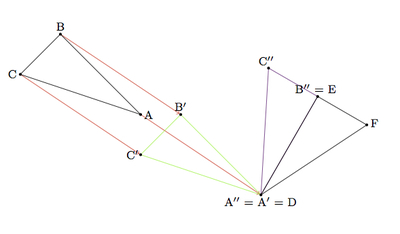# Why does SSS work?

Alignments to Content Standards: G-CO.B.8

In the picture below segment $AB$ is congruent to segment $DE$, segment $AC$ is congruent to segment $DF$ and segment $BC$ is congruent to segment $EF$:Show that the two triangles $ABC$ and $DEF$ are congruent via the following steps, which produce a rigid transformation of the plane sending $\triangle ABC$ to $\triangle DEF$.

1. Show that there is a translation of the plane which maps $A$ to $D$. Call $B'$ and $C'$ the images of $B$ and $C$ under this transformation.

2. Show that there is a rotation of the plane which does not move $D$ and which maps $B'$ to $E$. Call $C''$ the image of $C'$ under this transformation.

3. Show that there is a reflection of the plane which does not move $D$ or $E$ and which maps $C''$ to $F$.

## IM Commentary

This particular sequence of transformations which exhibits a congruency between triangles $ABC$ and $DEF$ used one translation, one rotation, and one reflection. There are many other ways in which to exhibit the congruency and students and teachers are encouraged to explore the different possibilities.

For example, in the first step, where point $A$ is moved via rigid transformation to point $D$, this could be accomplished via a translation, rotation, or reflection. The second step, where $B^\prime$ is moved to $E$ while leaving $D$ fixed, could be accomplished with a rotation as is done here or with a reflection.

It is important to note that the argument here relies on the SAS congruence criterion. An alternate approach to SSS which does not use SAS can be found here: https://www.illustrativemathematics.org/tasks/1930.

This task includes an experimental GeoGebra worksheet, with the intent that instructors might use it to more interactively demonstrate the relevant content material. The file should be considered a draft version, and feedback on it in the comment section is highly encouraged, both in terms of suggestions for improvement and for ideas on using it effectively. The file can be run via the free online application GeoGebra, or run locally if GeoGebra has been installed on a computer.

This file is designed to illustrate a series of rigid transformations to show two triangles are congruent. It contains instructions and at every step there is a button that can be clicked to show the next step. Also there are sliders that appear so you can manually do the rigid transformations. At any point you can click the red reset button to return to the initial state.

## Attached Resources

• 110 GeoGebra File
• ## Solution

1. Translation by the segment $AD$ maps $A$ to $D$. The displacement is shown by the red arrows in the picture below:The result of the translation is $\triangle A^\prime B^\prime C^\prime$ shown in green.

2. In order to move $B'$ to $E$, without moving $D$, we rotate triangle $A'B'C'$ about point $D$ by angle $B'A'E$, the effect of which is pictured below (the point $C''$ is the image of $C'$ under this rotation):We know that the image of $B'$, under rotation about $D$ by angle $B'A'E$, will be $E$ because, by assumption, $\overline{AB}$ is congruent to $\overline{DE}$.

3. Now it remains to move $C''$ to $F$, without moving $D$ or $E$. The only possible rigid motion of the plane other than the identity which does not move $D$ or $E$, is reflection about $\overleftrightarrow{DE}$ so we must check that this reflection moves $C^{\prime \prime}$ to $F$. We know that $|DF| = \left|A^{\prime \prime}C^{\prime \prime}\right|$ because translations and rotations preserve distances and $|AC| = |DF|$. Similar reasoning shows that $|EF| = \left|B^{\prime \prime}C^{\prime \prime}\right|$. Hence $\triangle DFC^{\prime \prime}$ is isosceles with $m(\angle DFC^{\prime \prime}) = m(\angle DC^{\prime \prime}F)$. Similarly $m(\angle EFC^{\prime \prime}) = m(\angle EC^{\prime \prime} F)$. This means that $m(\angle F) = m(\angle C^{\prime \prime})$. By SAS $\triangle DEF$ is congruent to $\triangle DB^{\prime \prime}C^{\prime \prime}$. This means that $\overleftrightarrow{DE}$ is the perpendicular bisector of $\overline{C^{\prime \prime}F}$. Reflection over $\overleftrightarrow{C^{\prime \prime}F}$ maps $C^{\prime \prime}$ to $F$ and shows that $\triangle ABC$ is congruent to $\triangle DEF$.